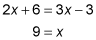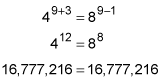##### Algebra II For Dummies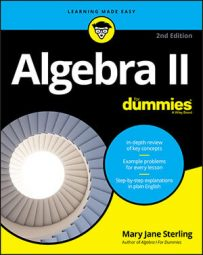You can use the base rule to solve algebraic equations with different bases, as long as the bases are related to one another by being powers of the same number.

If you have an equation written in the form bx = by, where the same number represents the bases b, the following rule holds: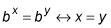You read the rule as follows: "If b raised to the xth power is equal to b raised to the yth power, that implies that x = y." The double-pointed arrow indicates that the rule is true in the opposite direction, too.

Using the base rule to solve the equation 23 + x = 24x – 9, you see that the bases (the 2s) are the same, so the exponents must also be the same. You just pull the exponents down and solve the linear equation 3 + x = 4x – 9 for the value of x: 12 = 3x, or x = 4. You then put the 4 back into the original equation to check your answer: 23 + 4 = 24(4) – 9, which simplifies to 27 = 27, or 128 = 128.

Many times, bases are related to one another by being powers of the same number. For example, to solve the equation 4x + 3 = 8x – 1, you need to write both the bases as powers of 2 and then apply the rules of exponents. Here are the steps of the solution:

1. Change the 4 and 8 to powers of 2.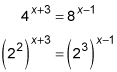2. Raise a power to a power.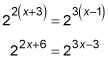3. Equate the two exponents, because the bases are now the same, and then solve for x.